## Vector Projection

Suppose we have a vector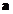in 2-dimensional space, and we would like to find the component of vectorin the direction of horizontal and vertical axis. Using trigonometry we can find the magnitude of vector component to be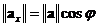and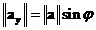. The direction of these vector components are the standard unit vector of horizontal and vertical axes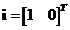and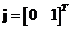.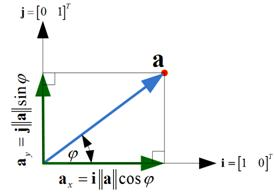We can find vector projection of a vectoronto other vector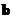based on the inner product of the two vectors. Scalar projection of vectoronto vectoris the magnitude of projection given as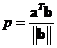. Vector projection of vectoronto vectoris the magnitude of projection times the unit vector ofbecomes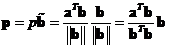. Geometrically, vector projection is shown in the figure below.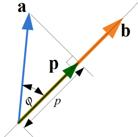Example
Projection of vector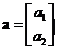onto horizontal axis is equivalent to projection of vectoronto unit standard vector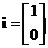. The scalar projection is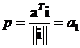. The projection vector becomes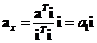. Cosine angle between two vectors is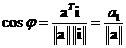. Thus,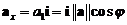.

The interactive program below will give you the projection vectors and the scalar projection. You need to provide 2 vectors as the input. Random Example button will generate random input vectors.

vector x vector y